### Solar Energy

About $99\%$ of the solar power reaching Earth's surface has a wavelength between $\SI{300}{\nano\meter}$ and $\SI{2.5}{\micro\meter}$.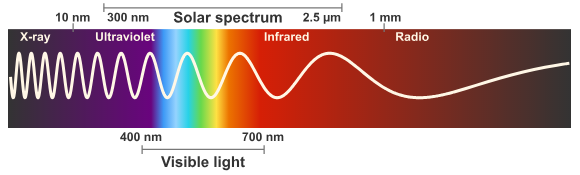While this sets limits for the wavelengths of solar photons, they still cover a wide range: solar photons can differ in wavelength by a factor of 10. This is significant because photons with different wavelengths can interact with us in very different ways:

• ultraviolet photons, with wavelengths shorter than $\SI{400}{\nano\meter}$, are responsible for giving us sunburns
• visible photons, with wavelengths from roughly $\SI{400 - 700}{\nano\meter}$, allow us to see
• infrared photons, with wavelengths longer than $\SI{700}{\nano\meter}$, make us feel warm

We would interpret $1000\SI[per-mode=symbol]{}{\watt\per\meter\squared}$ of these different types of radiation in very different ways, even though in each case it would be the same intensity of radiation. This wide range of solar wavelengths, as well as their distribution (i.e. which wavelengths are most common), is important in analyzing many solar energy conversion technologies.

# Spectral Properties of Sunlight

Which wavelengths make up the peak intensity of solar radiation?Hint: keep in mind that life on Earth evolved to be sensitive to electromagnetic radiation with wavelengths it was most exposed to (i.e., the wavelengths with the highest intensity). If a particular type of energy or radiation isn't available on Earth, it can't influence what happens here.

# Spectral Properties of Sunlight

The solar spectrum at Earth’s surface is shown in the plot of spectral irradiance below. Higher values mean more solar energy comes in the form of photons with that wavelength. The peak intensity of solar radiation is in the visible spectrum, but there is still a significant amount of solar energy in ultraviolet and infrared wavelengths.Approximately $4\%$ of the solar energy reaching Earth's surface is in the ultraviolet spectrum, $44\%$ is in the visible spectrum, and $52\%$ is in the infrared spectrum.

When looking at the plot above, it's important to note that it shows spectral intensity rather than intensity. Intensity measures power per unit area, while spectral intensity measures power per unit area per unit wavelength. This distinction is important when we want to calculate the power contained within a certain band of wavelengths. For example, for the power contained in the visible spectrum, we only want to consider wavelengths from $\SI{400}{\nano\meter}$ to $\SI{700}{\nano\meter}$.

# Spectral Properties of Sunlight

From the Planck relation, we know that longer wavelengths correspond to lower photon energies.

Why does the peak intensity of the solar spectrum lie in the visible spectrum, when ultraviolet photons have shorter wavelengths (and therefore more energy)?# Spectral Properties of Sunlight

Approximately $44\%$ of the solar energy reaching Earth is in the visible spectrum, while about $52\%$ is in the infrared spectrum.How can there be more energy in the infrared spectrum when the peak intensity is in the visible portion of the spectrum?

# Spectral Properties of Sunlight

While photon energy, spectral intensity, and total power contained in a particular spectrum are all related, they are not equivalent. This is why, in the solar spectrum, ultraviolet photons have the highest energy, visible photons have the highest spectral intensity, but infrared photons deliver the most total energy.

We already learned that we can calculate intensity, which is power per unit area, from the product of photon energy and photon flux: $I = \Phi E$. However, that expression only holds if each photon has the same energy. For light that has a mix of photons with different energies, we would need to add up the contribution from each different wavelength: $I = \sum_\lambda \Phi_\lambda E_\lambda$, where $\Phi_\lambda$ is the flux of photons with the wavelength $\lambda$ and $E_\lambda$ is the energy of a photon with the wavelength $\lambda$. The product of $\Phi_\lambda$ and $E_\lambda$ is the incident power due to the flux of photons of a specific wavelength - in other words, it's the spectral intensity $I_\lambda$. This means we can also calculate total intensity from $I = \sum_\lambda I_\lambda$.

If we want to calculate the power contained in a certain wavelength range, we should just add up the contributions from photons in that wavelength range: $Q_{\lambda_1,\lambda_2} = \sum_{\lambda = \lambda_1}^{\lambda_2} I_{\lambda}$ Summing over the photons with wavelengths ranging from $\lambda_1$ to $\lambda_2$ is equivalent to taking an integral over that same range. So we can also calculate the total power in a certain spectral range by integrating the intensities over that range: $Q_{\lambda_1,\lambda_2} = \int_{\lambda_1}^{\lambda_2} I_{\lambda} d\lambda$

Graphically, calculating the integral of a function is equivalent to finding the area under the curve of that function. That means that a simple way of estimating the energy contained in different parts of the solar spectrum is to compare the areas of those parts of the spectrum. This matches the fact that roughly $4\%$, $44\%$, and $52\%$ of solar energy are contained in the ultraviolet, visible, and infrared spectra, respectively.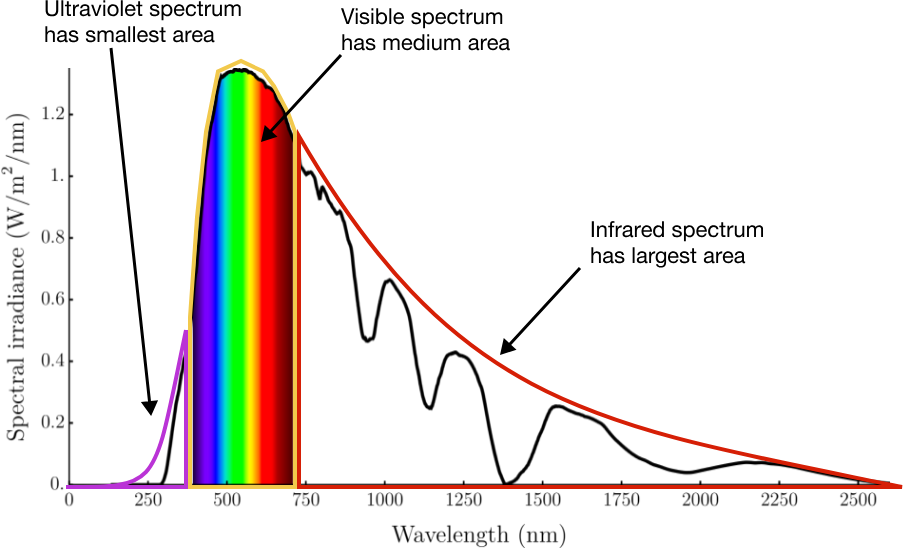# Spectral Properties of Sunlight

Suppose we have a solar collector which is only able to utilize photons that have an energy of at least $\SI{1.1}{\electronvolt}$. (PV cells work like this, which we'll learn more about in Chapter 4.)Approximately what portion of solar energy is carried by photons that have an energy of at least $\SI{1.1}{\electronvolt}$?

Recall that the Planck relation, which relates photon energy $E$ and wavelength $\lambda$, is: $E = \frac{hc}{\lambda}$ where the product of the Planck constant and the speed of light is given by $hc = \SI{1240}{\electronvolt\nano\meter}.$

# Spectral Properties of Sunlight

The wide range of wavelengths in the solar spectrum is explained by the fact that sunlight originates as thermal radiation from the Sun. Everything emits thermal radiation, but the intensity and wavelength distribution of that radiation depends on its temperature.

A glowing coal is hot enough to emit some visible radiation, which is why it appears to glow, whereas most objects we encounter in everyday life only emit infrared radiation, which we cannot see.

A black body is an object that absorbs all incident radiation and emits the maximum amount of thermal radiation that any object can emit (for its given temperature). Objects at very high temperature tend to act like black bodies.

The spectrum of sunlight reaching Earth is pretty close to that of a perfect black body: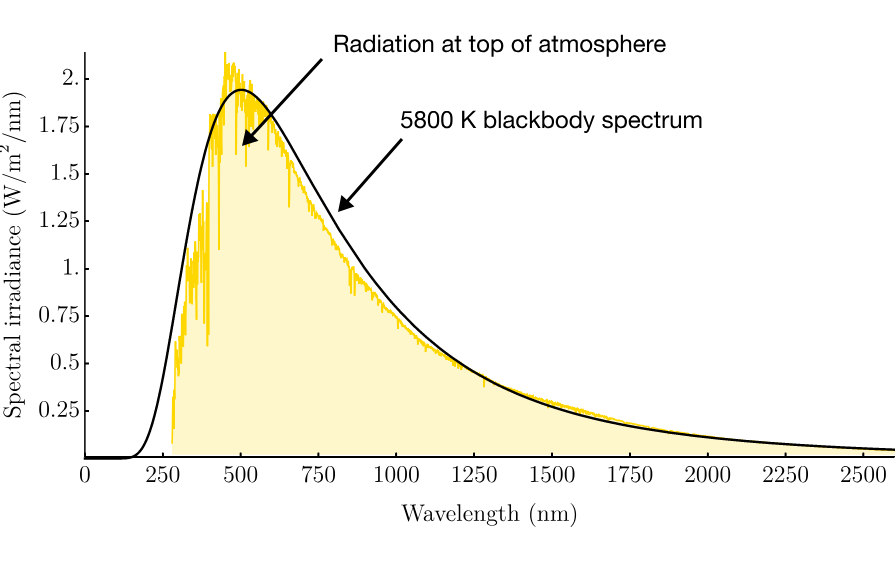To calculate the exact spectral distribution of thermal radiation for a black body, we can use Planck's law. The black body spectrum as given by Planck's law depends on the temperature $T$ (in Kelvin) of the black body according to

$\displaystyle B_{\lambda}(\lambda,T) = \frac{2\pi h c^2}{ \lambda^5}\frac{1}{e^{h c/\lambda k_B T}-1},$

where $B_{\lambda}$ is the spectral irradiance of the body in terms of wavelength $\lambda$, typically reported in units of $\si[per-mode=symbol]{\watt\per\meter\squared\per\nano\meter}$. Here $c = \SI[per-mode=symbol]{3e8}{\meter\per\second}$ is the speed of light, $h$ is the Planck constant (for this equation it is more useful to use standard SI units, in which case $h = \SI{6.626e-34}{\joule\second}),$ and $k_B = \SI[per-mode=symbol]{1.38e-23}{\joule\per\kelvin}$ is the Boltzmann constant.

# Spectral Properties of Sunlight

From Planck's law, we can see that a black body emitter will have a peak emission wavelength (a wavelength which corresponds to the highest spectral radiance for that emitter), which will be a function of the temperature of that emitter.

Recall Planck's law: $\displaystyle B_{\lambda}(\lambda,T) = \frac{2\pi h c^2}{\lambda^5}\frac{1}{e^{hc/\lambda k_B T}-1}$

You can find the relationship between the peak emission wavelength and temperature by taking the derivative of spectral irradiance with respect to wavelength and setting it to zero. This finds the maximum spectral irradiance value because it finds the point on the curve that corresponds to zero slope.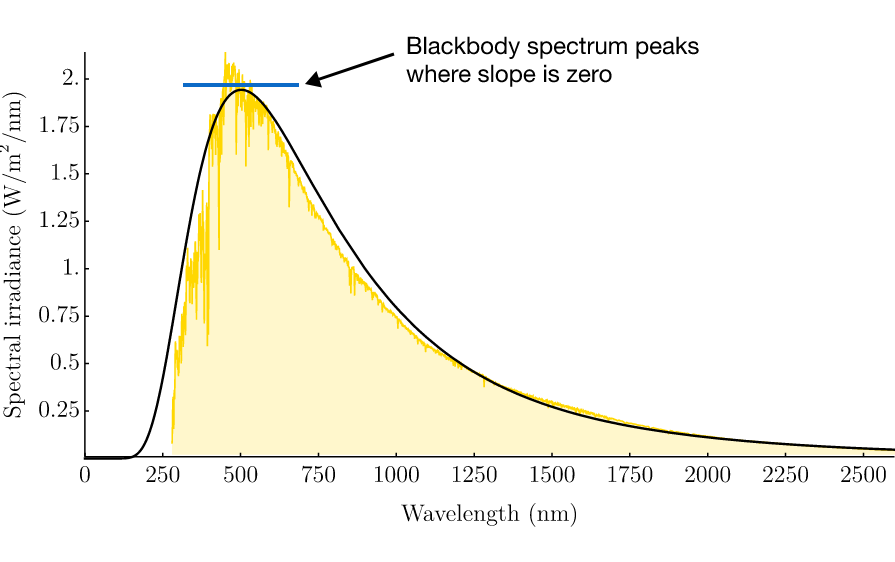The peak solar wavelength is around $\SI{500}{\nano\meter}$. What temperature (in Kelvin) corresponds to a black body with a peak emission wavelength of $\SI{500}{\nano\meter}$?

Remember:

• $c = \SI[per-mode=symbol]{3e8}{\meter\per\second}$
• $h = \SI{6.626e-34}{\joule\second}$
• $k_B = \SI[per-mode=symbol]{1.38e-23}{\joule\per\kelvin}$

Hint: The solution to $x e^x/\left(e^x-1\right)=5$ is $x \approx 4.965$. This should come in handy when you take the derivative of Planck's law with respect to wavelength and set it equal to zero.

# Spectral Properties of Sunlight

Solar photons have a wide range of wavelengths, and the solar spectral irradiance curve is characteristic of thermal radiation from a black body at $\SI{5800}{\kelvin}$. This is because Sunlight is thermal radiation from the Sun, which has a surface temperature of around $\SI{5800}{\kelvin}$.

The actual spectrum which reaches Earth’s surface looks a bit different from black body radiation from a $\SI{5800}{\kelvin}$ emitter, primarily due to absorption in the Earth's atmosphere. Certain gas species in the atmosphere absorb over narrow wavelength ranges, which leads to "missing" portions of the solar spectrum.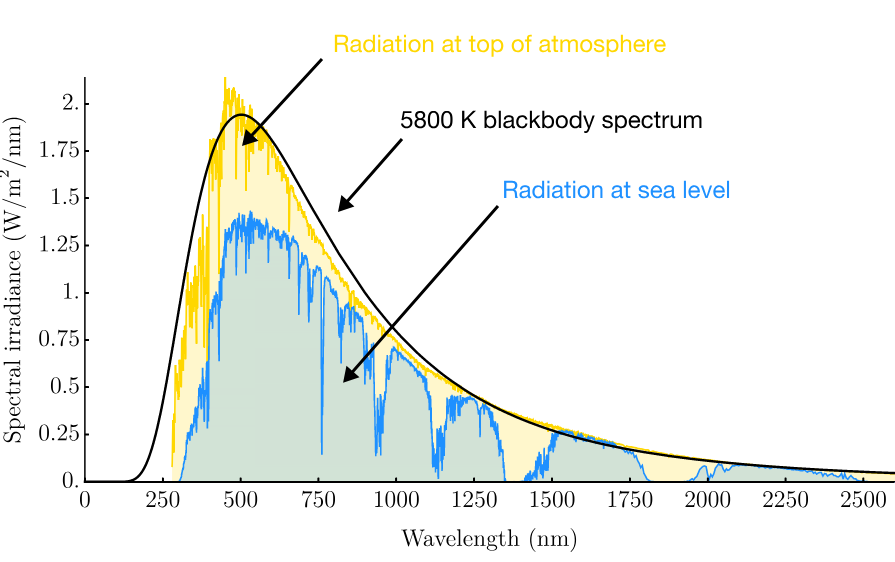This spectrum represents the distribution of wavelengths available in solar radiation. Many solar energy systems are optimized to convert this particular spectrum to electricity, since it represents the energy flow available to us on Earth. We'll learn about some of these systems in future chapters of this exploration.

# Spectral Properties of Sunlight

×